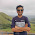## Sunday, May 16, 2010

### Irodov Problem 3.37

Similar to problem 3.34 we have,An equipotential surface is a surface over which the electric potential is exactly the same value. For example the equipotential surface around a point charge is a sphere. Here the equation of the surface is given by,We know that the equation of the circle is given by,where r is the radius of the circle. In other words we can generate the surface in (1) by simply staking circles on top of each other along the z-axis where the radii of these circles is given by ,In other words, if you take the curve,in the x-z plane and rotate it about the z-axis a complete circle we will obtain the surface in (1). This is shown in the figure.From basic conic sections we know that (3) results in either an ellipse or a hyperbola depending on the sign of b. Hence, by rotating these we would either get an ellipsoid or a hyperboloid.

#### 1 comment:

1.